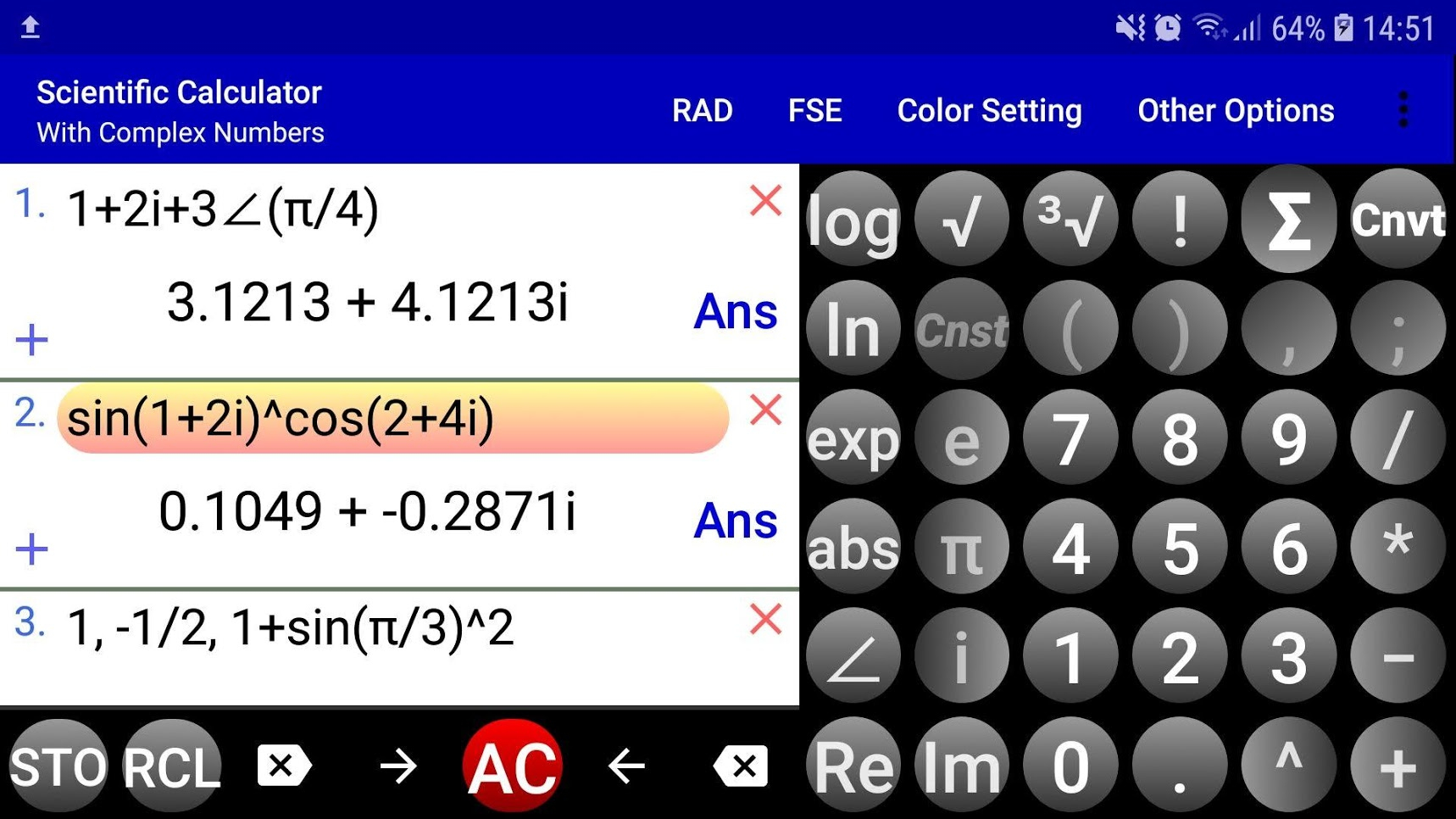Leider ist dieser Deal mittlerweile abgelaufen
108° Abgelaufen

# Complex Number Calculator | Scientific Calculator kostenlos (Android)

eingestellt am 28. Mär

### Dieser Deal ist leider abgelaufen. Hier sind ein paar andere Optionen für Dich:

Die App gibts derzeit kostenlos für alle Android Nutzer. Der Normalpreis liegt bei 3,49 €. Insgesamt wurde die App bereits über 50.000 mal runtergeladen und im Schnitt mit 4,2 / 5 Sternen vergeben.Integrated scientific calculator with complex number calculator to compute scientific/mathematical expressions containing real, imaginary and, in general, complex numbers in standard (rectangular) a+bi and polar (phasor) r∠(θ) forms such as (1/2 + 2i )(3 + 4i) + 3∠(π/4) + sin(i)cos(2 + 3i)^(4 - 2i)

The calculations can be performed in RAD (radians) or DEG (degrees) mode.

This comprehensive complex number calculator optionally converts the result or any complex number in standard form to polar and other modular forms and vice versa (long press i or ∠ to see all forms of the calculated result).

The result can be displayed with fixed, scientific and engineering notations.

This scientific calculator supports all main mathematical operations (addition, subtraction, multiplication and division) and functions (power, exponent, logarithm, trigonometric, hyperbolic and their inverses, etc.) with real, imaginary or complex numbers as their arguments.

Additionally, this scientific complex number calculator supports the Gamma function, Γ, and the psi function, Ψ, and the zeta function, ζ, with real/imaginary/complex numbers as their arguments.
Zusätzliche Info

### Gruppen

1 Kommentar
Dein Kommentar@
Text